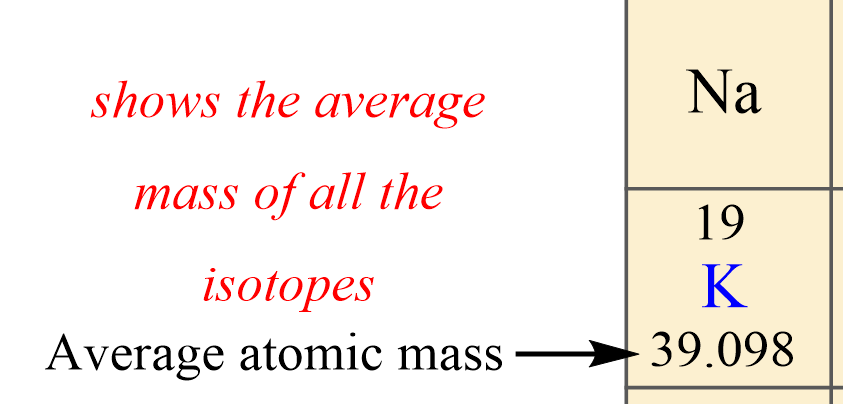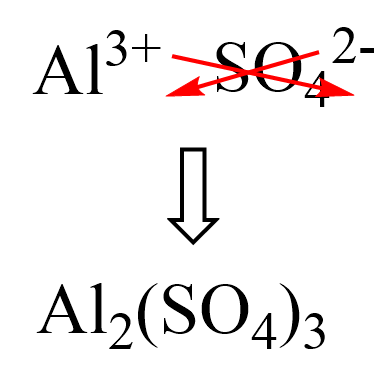## General Chemistry

The mass of one mole of atoms/molecules/ions is called its molar mass (M) expressed in g/mol. To calculate the molar mass of an atom or a molecule, we are always going to need a periodic table. So, how do we find the molar mass in the periodic table?

For example, there are two numbers given for potassium in the periodic table: 19 and 39.098. The smaller one is the atomic number which shows the number of protons, and the larger one is the average atomic mass:What’s important for today’s topic is that numerically, the molar mass is equal to the average atomic mass of the given atom, so we can look it up in the periodic table. For potassium then, it is 39.098 g/mol which means one mole of potassium atoms weighs 39.098 g. And we can do this for all the elements in the periodic table.

If there is a subscript in the symbol, then you need to multiply the molar mass by that number. For example, to find the molar of P4, we multiply 30.974 g/mol by 4:

M (P4) = 30.974 g/mol x 4 = 123.896 g/mol

Usually, we round off the molar mass to 1 or 2 decimal places, so for P, we’d use 123.9 or 123.90 for its molar mass.

# The Molar Mass of Molecules

To calculate the molar mass of a molecule, we add the molar mass of each constituent atom by the corresponding subscript.

For example, the molar mass of water would be:

M (H2O) = 2 x 1.0 + 16.0 = 18.0 g/mol

If the formula of the molecule is not given, you will need to first determine it.

For example, determine the molar mass of phosphorous pentachloride.

The formula of phosphorous pentachloride is PCl5. Go over naming covalent compounds to refresh some of the concepts here.

To calculate the molar mass of PCl5, we multiply the molar mass of each element by its subscript:

M (PCl5) = M (P) + 5x M (Cl)

M (PCl5) = 30.1 + 5 x 35.5 = 207.6 g/mol

Another example: what is the molar mass of aluminum sulfate?

First, we know that the aluminum ion has a 3+ charge. You need to know the common polyatomic ions from where we find that sulfate is the SO42- ion. Next, we write the ions next to each other and add the correct subscripts:And now, we multiply the molar mass of each element by its subscript. Remember, the number outside of parenthesis applies to all the elements in it.

M (Al2(SO4)3) = 2 x M (Al) + 3 x M (S) + 3 x 4 x M (O)

M (Al2(SO4)3) = 2 x 27.0 + 3 x 32.1 + 3 x 4 x 16.0 = 342.3 g/mol

# The Molar Mass of Ions

Do not worry about the charge of the ion. It is a result of either losing or gaining an electron(s) which has negligible mass compared to atoms and molecules.

For example, what is the molar mass of the nitrate ion?

The formula of nitrate ion is NO3, therefore, the molar mass would be:

M (NO3) = M (N) + 3 x M (O) = 14.0 + 3 x 16.0 = 62.0 g/mol

# The Molar Mass of Hydrates

To calculate the molar mass of a hydrate, determine the molar mass of the salt and add to the molar mass of the water multiplied by its subscript.

For example, calculate the molar mass of nickel (II) chloride hexahydrate.

He formula of the hydrate is NiCl2·6H2O, and therefore, the molar mass would be:

M (NiCl2·6H2O) = M (Ni) + 2 M (Cl) + 6 M (H2O)

M = 58.7 + 2 x 35.5 + 6 x 18.0 = 237.7 g/mol

Calculating the molar mass is essential as it lays the foundation for most of the calculations in stoichiometry such as the mass, moles, number of particles and etc.

Check Also